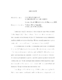## Combinatorics of K-Theoretic Jeu de Taquin2010
##### Authors
Clifford, Edward Grant
Thomas and Yong  introduced a theory of jeu de taquin which extended Schutzenberger's  for Young tableaux. The extended theory computes structure constants for the K-theory of (type A) Grassmannians using combinatorial machinery similar to that for cohomology. This rule naturally generalizes to give a conjectural root-system uniform rule for any minuscule flag variety G/P. In this dissertation, we see that the root-system uniform rule is well-defined for certain G/P other than the Grassmannian. This gives rise to combinatorially defined rings which are conjecturally isomorphic to K(G/P). Although we do not prove that these rings are isomorphic to K(G/P), we do produce a Pieri rule" for computing the product of a general class with a generating class in the type B combinatorial case. We also investigate some symmetries which support the conjectural isomorphism. Moreover, our results combined with recent work of Buch and Ravikumar  imply that this conjecture is in fact true. Lenart  gave a Pieri rule for the type A K-theory, demonstrating that the Pieri structure constants are binomial coefficients. In contrast, using techniques of , we show that type B Pieri structure constants have no such simple closed forms. References:  A. Buch and V. Ravikumar: Pieri rules for the K-theory of cominuscule Grassmannians, arXiv:1005.2605, 2010.  C. Lenart: Combinatorial aspects of K-theory of Grassmannians. Ann. Combin. 4 (2000), 67--82.  M. Petkovsek and H. Wilf and D. Zeilberger: A=B. A K Peters, Ltd. (1996).  M.-P. Schutzenberger: Combinatoire et representation du groupe symetrique. Springer-Verlag Berlin, Lec. Notes in Math. 579 (1977), 59--113.  H. Thomas and A. Yong: A jeu de taquin theory for increasing tableaux, with applications to K-theoretic Schubert calculus. Algebra Number Theory 3 (2009), no. 2, 121--148.